# VS2022输出不了图片，同时显示找不到.NET Framework4.0

###### 问题遇到的现象和发生背景 新学期新课，学了python，假期尝试自己调试代码，用的VS2022，不知道为什么会这样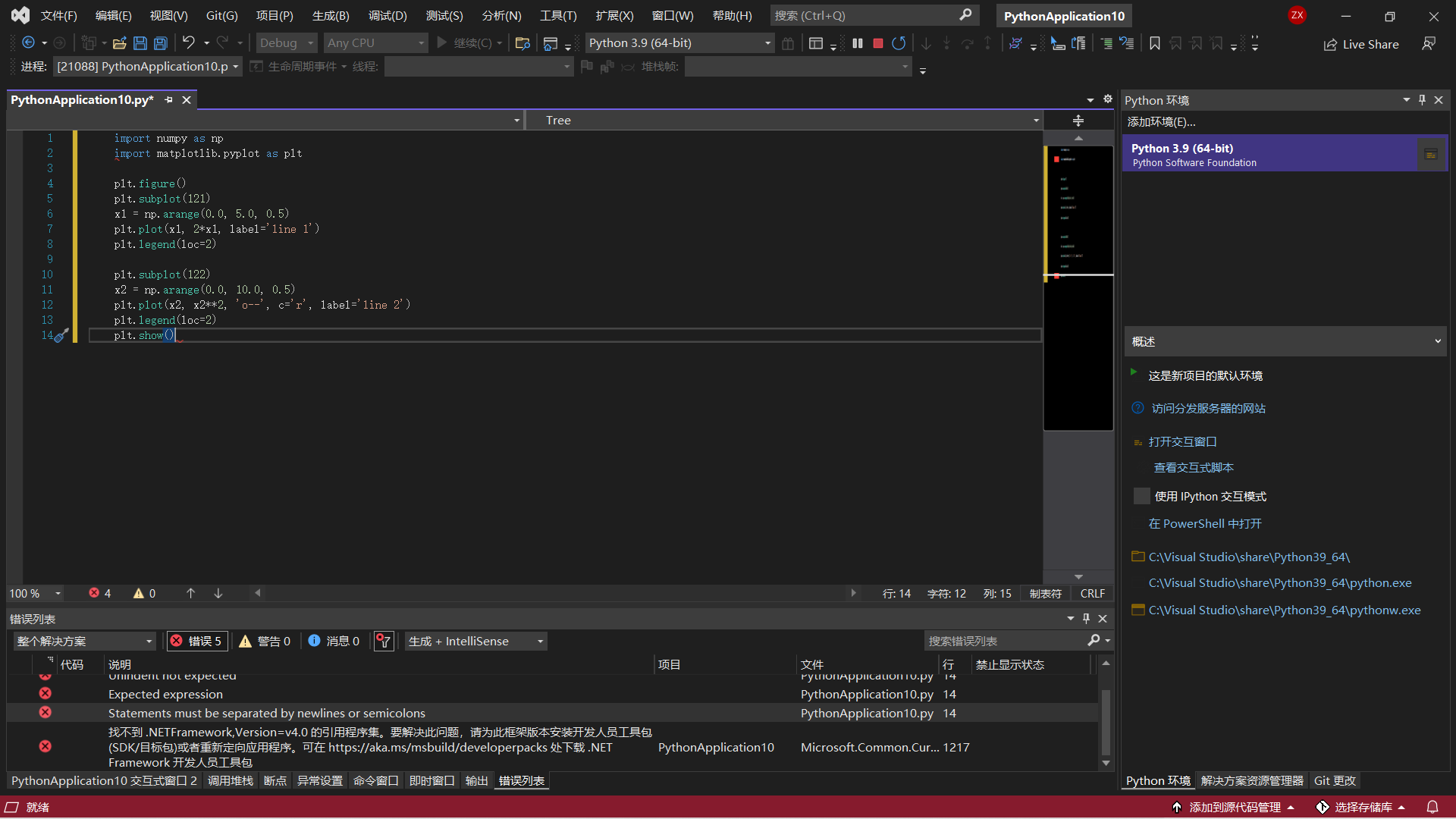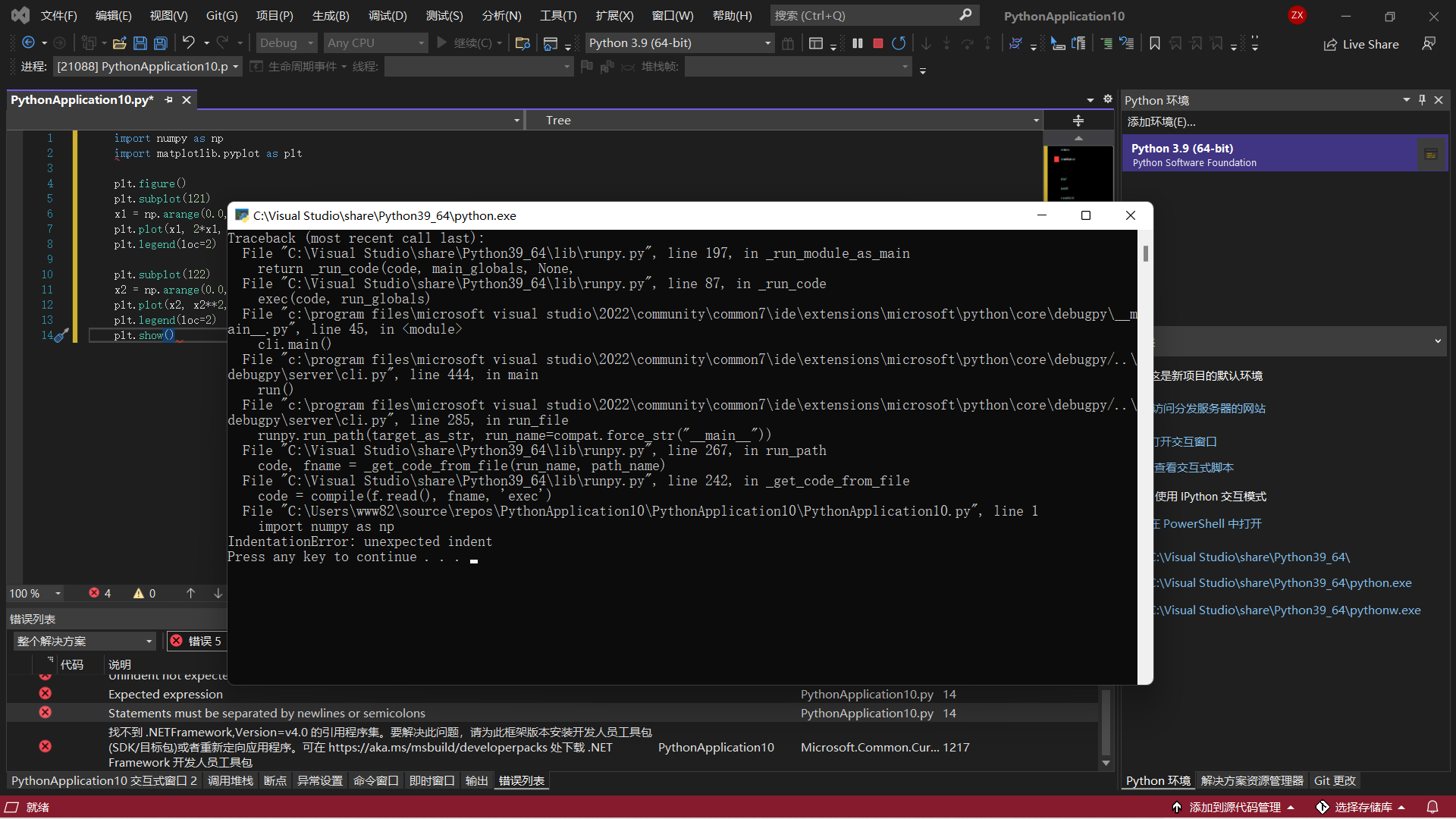• 写回答

#### 2条回答默认 最新

•CSDN专家-showbo 2022-01-28 21:26
关注

缩进有问题，文章里面的代码复制粘贴后前面有空白，全部去掉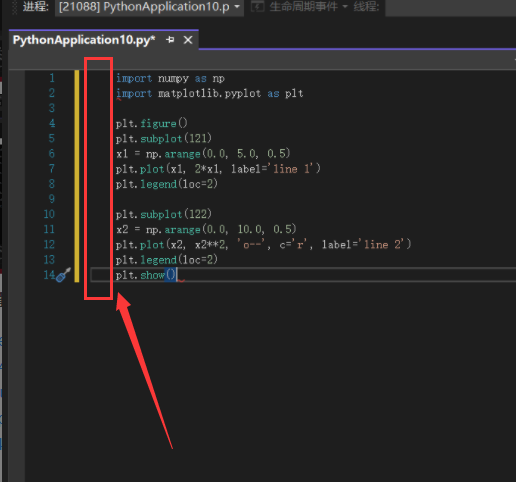或者复制下面的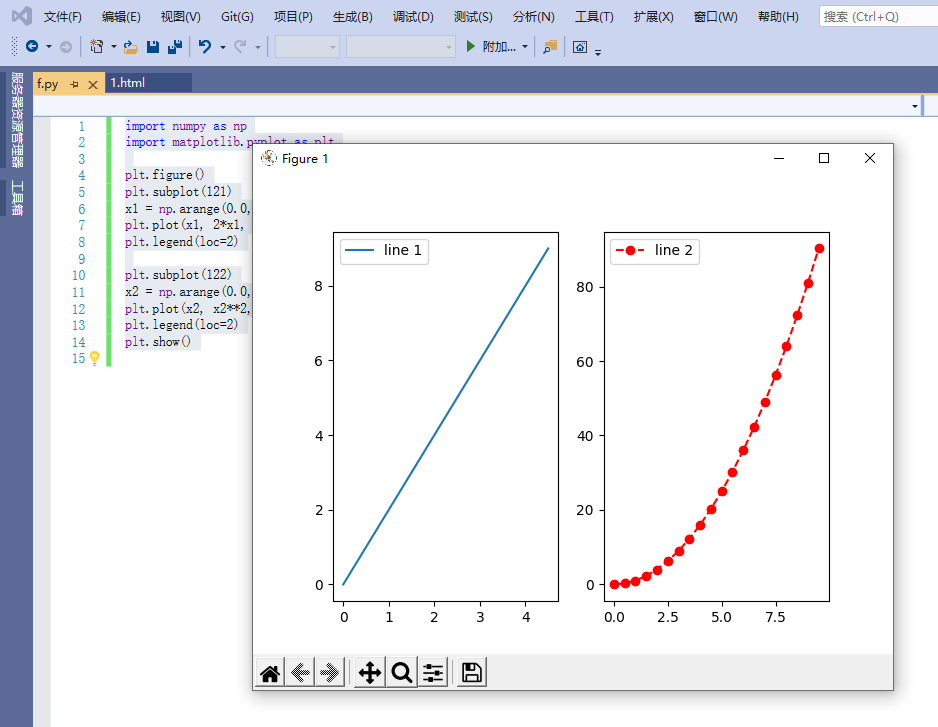``````import numpy as np
import matplotlib.pyplot as plt

plt.figure()
plt.subplot(121)
x1 = np.arange(0.0, 5.0, 0.5)
plt.plot(x1, 2*x1, label='line 1')
plt.legend(loc=2)

plt.subplot(122)
x2 = np.arange(0.0, 10.0, 0.5)
plt.plot(x2, x2**2, 'o--', c='r', label='line 2')
plt.legend(loc=2)
plt.show()

``````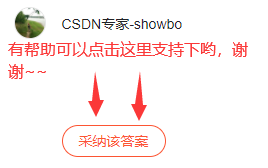本回答被题主选为最佳回答 , 对您是否有帮助呢?
评论

#### 悬赏问题

• ¥15 关于网上一个easyx制作的见缝插针小游戏(c++)
• ¥15 开地址法双散列函数处理碰撞
• ¥15 想问一下这个是什么情况 虚拟机Linux打不开了
• ¥15 联通光猫掉注册了怎么重新注册上去
• ¥15 关于unity开发steamvr程序遇到的问题